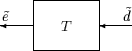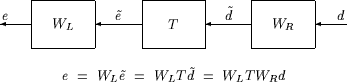## Interpretation of H-Infinity Norm

### Norms of Signals and Systems

There are several ways of defining norms of a scalar signal e(t) in the time domain. We will often use the 2-norm, (L2-norm), for mathematical convenience, which is defined as

`${‖e‖}_{2}:={\left({\int }_{-\infty }^{\infty }e{\left(t\right)}^{2}dt\right)}^{\frac{1}{2}}.$`

If this integral is finite, then the signal e is square integrable, denoted as e ∊ L2. For vector-valued signals

`$e\left(t\right)=\left[\begin{array}{c}{e}_{1}\left(t\right)\\ {e}_{2}\left(t\right)\\ ⋮\\ {e}_{n}\left(t\right)\end{array}\right],$`

the 2-norm is defined as

`$\begin{array}{c}{‖e‖}_{2}:={\left({\int }_{-\infty }^{\infty }{‖e\left(t\right)‖}_{2}^{2}dt\right)}^{\frac{1}{2}}\\ ={\left({\int }_{-\infty }^{\infty }{e}^{T}\left(t\right)e\left(t\right)dt\right)}^{\frac{1}{2}}.\end{array}$`

In µ-tools the dynamic systems we deal with are exclusively linear, with state-space model

`$\left[\begin{array}{c}\stackrel{˙}{x}\\ e\end{array}\right]=\left[\begin{array}{cc}A& B\\ C& D\end{array}\right]\left[\begin{array}{c}x\\ d\end{array}\right],$`

or, in the transfer function form,

e(s) = T(s)d(s), T(s):= C(sI – A)–1B + D

Two mathematically convenient measures of the transfer matrix T(s) in the frequency domain are the matrix H2 and H norms,

`$\begin{array}{l}{‖T‖}_{2}:={\left[\frac{1}{2\pi }{\int }_{-\infty }^{\infty }{‖T\left(j\omega \right)‖}_{F}^{2}d\omega \right]}^{\frac{1}{2}}\\ {‖T‖}_{\infty }:=\underset{\omega \in R}{\mathrm{max}\overline{\sigma }}\left[T\left(j\omega \right)\right],\end{array}$`

where the Frobenius norm (see the MATLAB® `norm` command) of a complex matrix M is

`${‖M‖}_{F}:=\sqrt{\text{Trace}\left({M}^{*}M\right)}.$`

Both of these transfer function norms have input/output time-domain interpretations. If, starting from initial condition x(0) = 0, two signals d and e are related by

`$\left[\begin{array}{c}\stackrel{˙}{x}\\ e\end{array}\right]=\left[\begin{array}{cc}A& B\\ C& D\end{array}\right]\left[\begin{array}{c}x\\ d\end{array}\right],$`

then

• For d, a unit intensity, white noise process, the steady-state variance of e is ∥T2.

• The L2 (or RMS) gain from d → e,

`$\underset{d\ne 0}{\mathrm{max}}\frac{{‖e‖}_{2}}{{‖d‖}_{2}}$`

is equal to ∥T. This is discussed in greater detail in the next section.

### Using Weighted Norms to Characterize Performance

Any performance criterion must also account for

• Relative magnitude of outside influences

• Frequency dependence of signals

• Relative importance of the magnitudes of regulated variables

So, if the performance objective is in the form of a matrix norm, it should actually be a weighted norm

WLTWR

where the weighting function matrices WL and WR are frequency dependent, to account for bandwidth constraints and spectral content of exogenous signals. The most natural (mathematical) manner to characterize acceptable performance is in terms of the MIMO ∥·∥ (H) norm. For this reason, this section now discusses some interpretations of the H norm.

Unweighted MIMO SystemSuppose T is a MIMO stable linear system, with transfer function matrix T(s). For a given driving signal $\stackrel{˜}{d}\left(t\right)$, define $\stackrel{˜}{e}$ as the output, as shown below.

Note that it is more traditional to write the diagram in Unweighted MIMO System: Vectors from Left to Right with the arrows going from left to right as in Weighted MIMO System.

Unweighted MIMO System: Vectors from Left to RightThe two diagrams shown above represent the exact same system. We prefer to write these block diagrams with the arrows going right to left to be consistent with matrix and operator composition.

Assume that the dimensions of T are ne × nd. Let β > 0 be defined as

`$\beta :={‖T‖}_{\infty }:=\underset{\omega \in R}{\mathrm{max}\overline{\sigma }\left[T\left(j\omega \right)\right]}.$`

Now consider a response, starting from initial condition equal to 0. In that case, Parseval's theorem gives that

`$\frac{{‖\stackrel{˜}{e}‖}_{2}}{{‖\stackrel{˜}{d}‖}_{2}}=\frac{{\left[{\int }_{0}^{\infty }{\stackrel{˜}{e}}^{T}\left(t\right)\text{\hspace{0.17em}}\stackrel{˜}{e}\left(t\right)dt\right]}^{\frac{1}{2}}}{{\left[{\int }_{0}^{\infty }{\stackrel{˜}{d}}^{T}\left(t\right)\text{\hspace{0.17em}}\stackrel{˜}{d}\left(t\right)dt\right]}^{\frac{1}{2}}}\le \beta .$`

Moreover, there are specific disturbances d that result in the ratio ${‖\stackrel{˜}{e}‖}_{2}/{‖\stackrel{˜}{d}‖}_{2}$ arbitrarily close to β. Because of this, ∥T is referred to as the L2 (or RMS) gain of the system.

As you would expect, a sinusoidal, steady-state interpretation of ∥T is also possible: For any frequency $\overline{\omega }\in R$, any vector of amplitudes $a\in {R}_{{n}_{d}}$, and any vector of phases $\varphi \in {R}^{{n}_{d}}$, with ∥a2 ≤ 1, define a time signal

`$\stackrel{˜}{d}\left(t\right)=\left[\begin{array}{c}{a}_{1}\mathrm{sin}\left(\overline{\omega }t+{\varphi }_{1}\right)\\ ⋮\\ {a}_{{n}_{d}}\mathrm{sin}\left(\overline{\omega }t+{\varphi }_{{n}_{d}}\right)\end{array}\right].$`

Applying this input to the system T results in a steady-state response ${\stackrel{˜}{e}}_{ss}$ of the form

`${\stackrel{˜}{e}}_{ss}\left(t\right)=\left[\begin{array}{c}{b}_{1}\mathrm{sin}\left(\overline{\omega }t+{\varphi }_{1}\right)\\ ⋮\\ {b}_{{n}_{e}}\mathrm{sin}\left(\overline{\omega }t+{\varphi }_{{n}_{e}}\right)\end{array}\right].$`

The vector $b\in {R}^{{n}_{e}}$ will satisfy ∥b2 ≤ β. Moreover, β, as defined in Weighted MIMO System, is the smallest number such that this is true for every ∥a2 ≤ 1, $\overline{\omega }$, and ϕ.

Note that in this interpretation, the vectors of the sinusoidal magnitude responses are unweighted, and measured in Euclidean norm. If realistic multivariable performance objectives are to be represented by a single MIMO ∥·∥ objective on a closed-loop transfer function, additional scalings are necessary. Because many different objectives are being lumped into one matrix and the associated cost is the norm of the matrix, it is important to use frequency-dependent weighting functions, so that different requirements can be meaningfully combined into a single cost function. Diagonal weights are most easily interpreted.

Consider the diagram of Weighted MIMO System, along with Unweighted MIMO System: Vectors from Left to Right.

Assume that WL and WR are diagonal, stable transfer function matrices, with diagonal entries denoted Li and Ri.

`${W}_{L}=\left[\begin{array}{cccc}{L}_{1}& 0& \dots & 0\\ 0& {L}_{2}& \dots & 0\\ ⋮& ⋮& \ddots & ⋮\\ 0& 0& \dots & {L}_{{n}_{e}}\end{array}\right],\text{ }{W}_{R}=\left[\begin{array}{cccc}{R}_{1}& 0& \dots & 0\\ 0& {R}_{2}& \dots & 0\\ ⋮& ⋮& \ddots & ⋮\\ 0& 0& \dots & {R}_{{n}_{d}}\end{array}\right].$`

Weighted MIMO SystemBounds on the quantity ∥WLTWR will imply bounds about the sinusoidal steady-state behavior of the signals $\stackrel{˜}{d}$and $\stackrel{˜}{e}\left(=T\stackrel{˜}{d}\right)$ in the diagram of Unweighted MIMO System: Vectors from Left to Right. Specifically, for sinusoidal signal $\stackrel{˜}{d}$, the steady-state relationship between $\stackrel{˜}{e}\left(=T\stackrel{˜}{d}\right)$, $\stackrel{˜}{d}$ and ∥WLTWR is as follows. The steady-state solution ${\stackrel{˜}{e}}_{ss}$, denoted as

 ${\stackrel{˜}{e}}_{ss}\left(t\right)=\left[\begin{array}{c}{\stackrel{˜}{e}}_{1}\mathrm{sin}\left(\overline{\omega }t+{\varphi }_{1}\right)\\ ⋮\\ {\stackrel{˜}{e}}_{{n}_{e}}\mathrm{sin}\left(\overline{\omega }t+{\varphi }_{{n}_{d}}\right)\end{array}\right]$ (1)

satisfies

`$\sum _{i=1}^{{n}_{e}}{|{W}_{{L}_{i}}\left(j\overline{\omega }\right){\stackrel{˜}{e}}_{i}|}^{2}\le 1$`

for all sinusoidal input signals $\stackrel{˜}{d}$ of the form

 $\stackrel{˜}{d}\left(t\right)=\left[\begin{array}{c}{\stackrel{˜}{d}}_{1}\mathrm{sin}\left(\overline{\omega }t+{\varphi }_{1}\right)\\ ⋮\\ {\stackrel{˜}{d}}_{{n}_{e}}\mathrm{sin}\left(\overline{\omega }t+{\varphi }_{{n}_{d}}\right)\end{array}\right]$ (2)

satisfying

`$\sum _{i=1}^{{n}_{d}}\frac{{|{\stackrel{˜}{d}}_{i}|}^{2}}{{|{W}_{{R}_{i}}\left(j\overline{\omega }\right)|}^{2}}\le 1$`

if and only if ∥WLTWR ≤ 1.

This approximately (very approximately — the next statement is not actually correct) implies that ∥WLTWR ≤ 1 if and only if for every fixed frequency $\overline{\omega }$, and all sinusoidal disturbances $\stackrel{˜}{d}$ of the form Equation 2 satisfying

`$|{\stackrel{˜}{d}}_{i}|\le |{W}_{{R}_{i}}\left(j\overline{\omega }\right)|$`

the steady-state error components will satisfy

`$|{\stackrel{˜}{e}}_{i}|\le \frac{1}{|{W}_{{L}_{i}}\left(j\overline{\omega }\right)|}.$`

This shows how one could pick performance weights to reflect the desired frequency-dependent performance objective. Use WR to represent the relative magnitude of sinusoids disturbances that might be present, and use 1/WL to represent the desired upper bound on the subsequent errors that are produced.

Remember, however, that the weighted H norm does not actually give element-by-element bounds on the components of $\stackrel{˜}{e}$ based on element-by-element bounds on the components of $\stackrel{˜}{d}$. The precise bound it gives is in terms of Euclidean norms of the components of $\stackrel{˜}{e}$ and $\stackrel{˜}{d}$ (weighted appropriately by WL(j$\overline{\omega }$) and WR(j$\overline{\omega }$)).